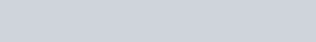# Power Series Distribution definition, formula with applications

A power series distribution is a discrete probability distribution that applies to a subset of natural numbers. The distribution’s names derive from the fact that the power series is used to construct them. This broad category of distributions includes a number of discrete distributions, such as the Poisson distribution, negative binomial distribution, and binomial distribution.

### Power Series Distribution

A discrete random variable X is said to have a generalized power series distribution if its probability function is given by,where f(θ) is a generating function and  f(θ) is positive finite and differentiable. Power series distribution is a discrete probability distribution.

### Properties

Some special properties of power series distribution are given-
• If θ=p/(1-p), f(θ)=(1+θ)^n and s={1,2,3,…,n), a set of (n+1) non-negative integers then the power series distribution is tends to binomial distribution.
• If f(θ)=e^θ and s={0,1,2,3,…,∞} then the distribution tends to poisson distribution.
• If θ=p/(1-p), f(θ)=(1+θ)^-n and s={0,1,2,3,…,∞), then the power series distribution tends to negative binomial distribution.
• If f(θ)=-log(1-θ) and s={1,2,….}, then the power series distribution tends to logarthmic distribution.

### Characteristics of power series distribution

Power Series Distribution to others distribution…